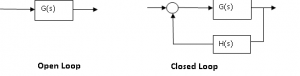Home > control systems > feedback control system

# Feedback Control System

The closed loop control systems are also called feedback control system. Figure shows the block diagram of closed loop(feedback control) system with more details the various terminologies used are as given below,

1. Reference Input /Command Input: The input to the entire system is called reference input.
2. Input transducer: This converts the form of the input to the form used by the controller
3. The output transducer: This measures the output response and converts it into the form used by the controller.
4. Feedback Path: It is the return path from the output to the summing junction. It consists of a feedback element which feeds back the output to the error detector
5. Error detector: The error detector receives the measured signal from output and compares it with the reference input. The difference of two signals produces the error signal also called as the actuating signal
6. Control Element/controller: it regulates the output according to the signal obtained from the error detector.Effect of Feedback on gain:

Consider a system with overall gain G(s) as shown in figure with feedback function H(S) then the overall gain of the system with negative feedback is given by G(s)/[1+ G(s)H(s)]. Hence for a negative feedback the gain G(S) is reduced by a factor = 1/[1+ G(s)H(s)]Effect of feedback on stability:

The feedback in a system can improve stability also it can be harmful if not applied properly

Effect of feedback on sensitivity:

A good control system should always be very insensitive to the parameter variations and it should be sensitive to commands

Hence feedback can increase or decrease the sensitivity of the system.

Effect of feedback on external disturbance or noise:

Feedback reduces the effect of noise and disturbances on the system performance.

In general the feedback also has effects on performance parameters such as bandwidth, impedance, transient response and frequency response, 1) Increases accuracy, 2)Increases the bandwidth and 3)Stabilizes the unstable system